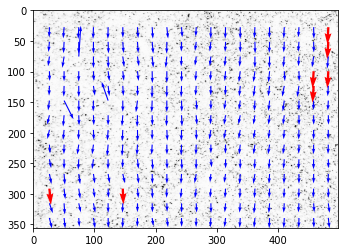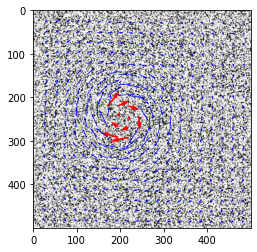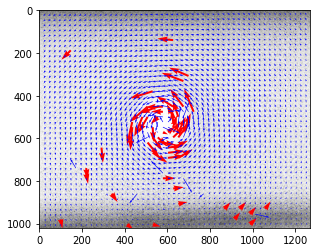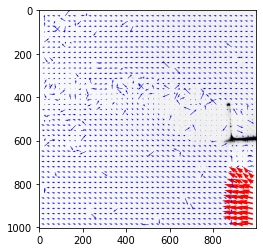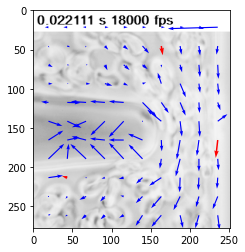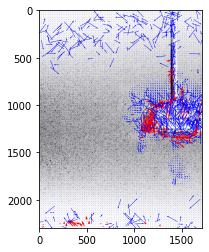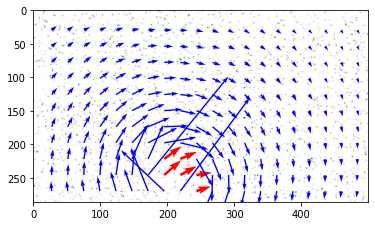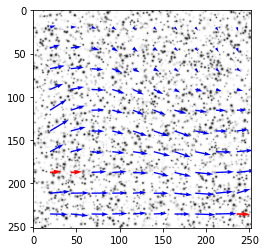## OpenPIV tutorial of all test cases¶

In :
from openpiv import tools, pyprocess, scaling, validation, filters
import numpy as np
import glob
%matplotlib inline

In :
%load_ext watermark
%watermark -v -m -p numpy,openpiv -g -b

CPython 3.8.5
IPython 7.19.0

numpy 1.19.4
openpiv 0.23.0a0

compiler   : GCC 7.3.0
system     : Linux
release    : 5.4.0-54-generic
machine    : x86_64
processor  : x86_64
CPU cores  : 8
interpreter: 64bit
Git hash   : 7d612c1a6b99c47b39947a349465bf0a203e7a60
Git branch : optional_normalize_intensity

In :
# set of typical parameters
window_size = 32  # pixels 32 x 32 pixels interrogation window, in frame A.
overlap = 16  # overlap is 8 pixels, i.e. 25% of the window
search_size = 40  # pixels 64 x 64 in frame B, avoids some peak locking for
# large displacements

In :
def openpiv_default_run(im1,im2):
""" default settings for OpenPIV analysis using
extended_search_area_piv algorithm for two images

Inputs:
im1,im2 : str,str = path of two image
"""

u, v, sig2noise = pyprocess.extended_search_area_piv(frame_a.astype(np.int32),
frame_b.astype(np.int32),
window_size=window_size,
overlap=overlap,
dt=1,
search_area_size=search_size,
sig2noise_method='peak2mean',
correlation_method='circular',
normalized_correlation=True)
x, y = pyprocess.get_coordinates(frame_a.shape,
search_size,
overlap)
# 5% lowest range
u, v, mask = validation.sig2noise_val(u, v,
sig2noise,
threshold = np.percentile(sig2noise,2.5))
u, v = filters.replace_outliers( u, v, method='localmean',
max_iter=3, kernel_size=3)
x, y, u, v = scaling.uniform(x, y, u, v, scaling_factor = 1. )
tools.save(x, y, u, v, sig2noise, mask, list_of_images+'.txt' )
fig,ax = tools.display_vector_field(list_of_images+'.txt',
on_img=True,image_name=list_of_images,
scaling_factor=1.,
ax=None)

In :
alist_filter = ['jpg','bmp','png','tif','tiff']

# all test cases in /openpiv/examples/
list_of_tests = glob.glob('../test*')
list_of_tests.sort()

list_of_images = []
for test in list_of_tests:

list_of_files = glob.glob(test+'/*.*')
list_of_files.sort()
list_of_images = [f for f in list_of_files if f[-3:] in alist_filter]
if len(list_of_images) > 0:
openpiv_default_run(list_of_images,list_of_images)